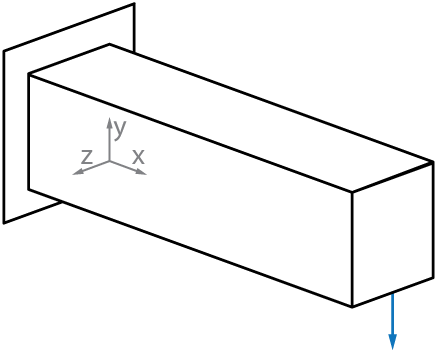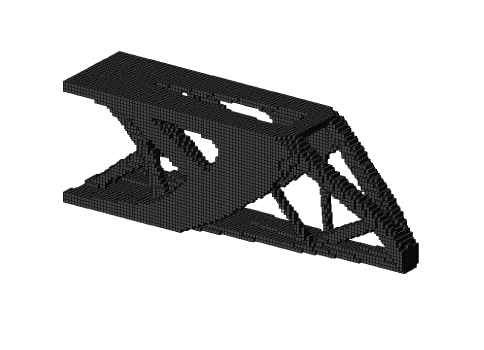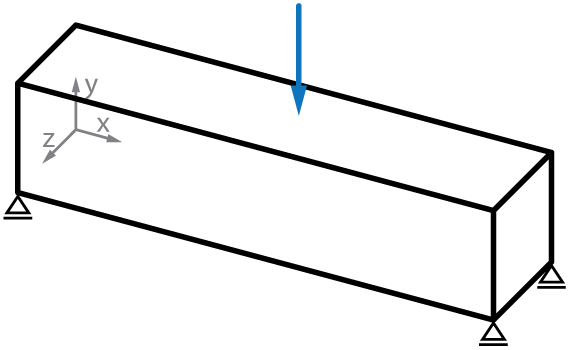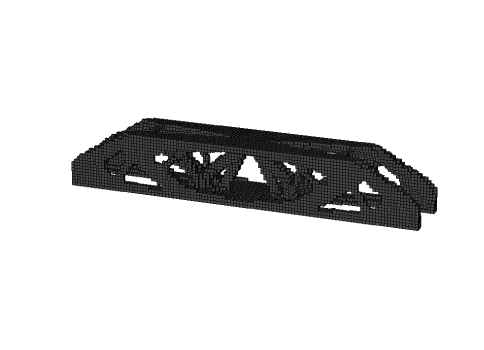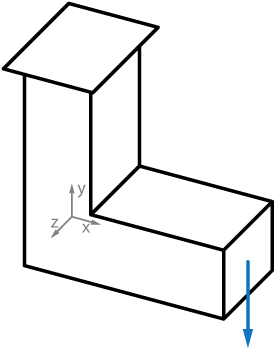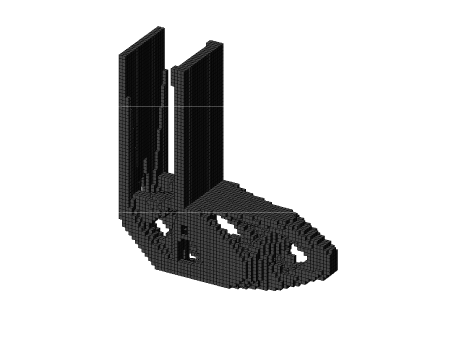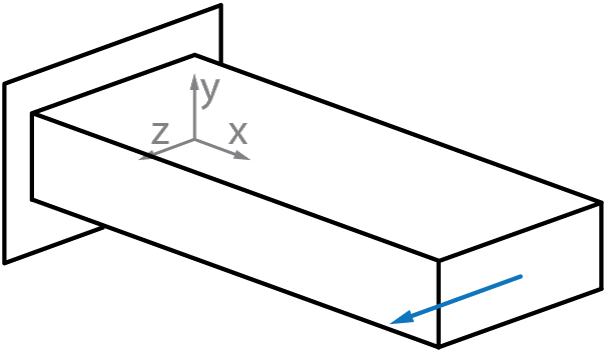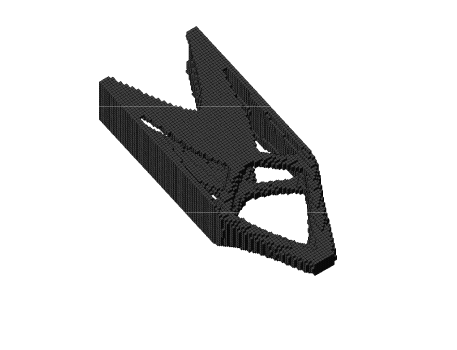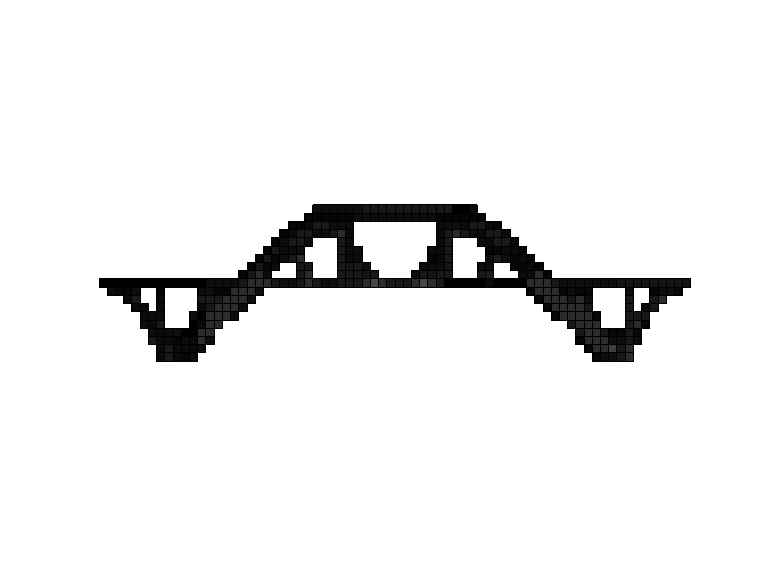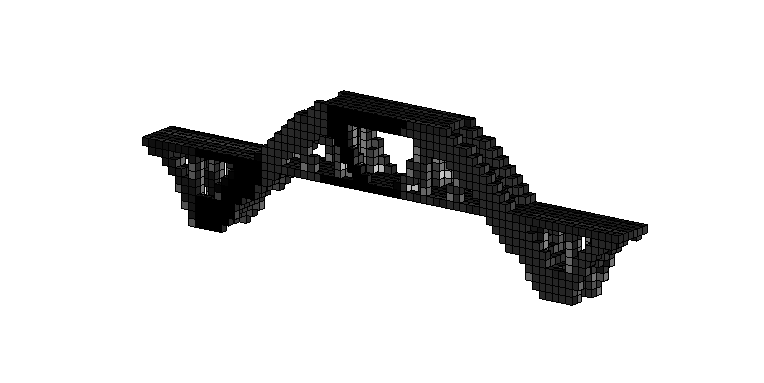# Iterative solver - Top3d

If the finite element mesh size becomes large, the traditional direct solver (line 72) used to address the finite element analysis is suffered by longer solving time and some other issues. However, iterative solver can solve large-scale problems efficiently. In this tutorial, you will learn how to replace direct solver by iterative solver in order to handle large-scale topology optimization problem.

To this end, line 72 is replaced by a built-in $\texttt{Matlab}$ function $\texttt{pcg}$, called preconditioned conjugate gradients method, as shown in the following

tolit = 1e-8;
maxit = 8000;
M = diag(diag(K(freedofs,freedofs)));
U(freedofs,:) = pcg(K(freedofs,freedofs),F(freedofs,:),tolit,maxit,M);


Direct solver is a special case by setting the pre-conditioner (line 74) to

M = inv(K);

Of course you can use other pre-conditioner such as incomplete choleksy by replace the code snippets above with the following

L = ichol(K(freedofs,freedofs));
U(freedofs,:) = pcg(K(freedofs,freedofs),F(freedofs,:),1e-8,1000,L,L');


The following are some large-scale problem we solved using top3d and iterative solver